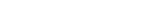MathExamle.com# Negative

Select from the list a generator that creates examples with answers and intermediate calculations that can be negative

### Conversant

Creates examples with addition and subtraction operations of integers, the answers and intermediate results can be negative

4 Variants 5 Levels
 -70+3= -46+56= -56-21= -30+40= -25+99= 78-17=
Variant: 1 Level: Normal

Creates expressions with comparisons of integers, the numbers can be negative

3 Variants 4 Levels
 -9-0 < 7+0 10+0 > 9-2 10-5 > 10-9 4+5 < 6+4
Variant: 2 Level: Easy

Creates linear equations, the answers are integers and can be negative

4 Variants 3 Levels
 -x×2=-8 x+4=-6 -2×x=-4 7÷x+1=-6 -x×2-10=6 -(-3+x)×3=6
Variant: A Level: Easy

Creates examples with operations of addition, subtraction, multiplication and division of integers, the answers can be negative

3 Variants 3 Levels
 -75+42÷3= -24×2-35= -29-24÷12= 8-32÷4= 25÷5+6= 52+80÷5=
Variant: 1 Level: Easy

Creates examples with operations of multiplication and division of negative and positive integers

4 Variants 4 Levels
 5×2= 70÷5= -7×8= -38÷2= -60÷(-3)= -66÷(-6)=
Variant: 1 Level: Easy

Creates examples with addition and subtraction operations of decimals, the answers can be negative

3 Variants 4 Levels
 0.05-45.9-49.15= -9.54-80.12-0.34= 59.44+16.07-80= -73.8-66+93.62=
Variant: 2 Level: Difficult

Creates examples with operations of addition, subtraction, multiplication and division of decimals, the answers can be negative

3 Variants 4 Levels
 -4.1×16+93.42= 54.72÷30-43.92= -84.456÷93.84+62.813= -1.25×2.24-37.2=
Variant: 1 Level: Difficult

### Denominator

Creates examples with addition and subtraction of fractions, the answers can be negative

3 Variants 3 Levels
 4 8 + 6 1 + 17 13 = 9 9 607
Variant: 2 Level: Hard

Creates expressions with comparisons of decimals, the numbers can be negative

3 Variants 4 Levels
 95 > -78 -96.706 < 88 -42.984 < -18.471 9.859 > -20.155
Variant: 1 Level: Difficult

Create expressions with comparisons of fractions, the numbers can be negative

3 Variants 3 Levels
 213 < 25 190 254 381
Variant: 1 Level: Hard

Creates examples with operations of addition, subtraction, multiplication and division of fractions, the answers can be negative

2 Variants 3 Levels
 ( – 1 – 1 ) ÷ 1 = 3 6 3
 – 1 × 5 1 – 15 = 6 4 8
Variant: A Level: Easy